# How to solve the integral which has limits from (1,2) to (2,4)

requied
Homework Statement:
Evaluate the integral below
Relevant Equations:
there is no equations(?)
I have a question like this;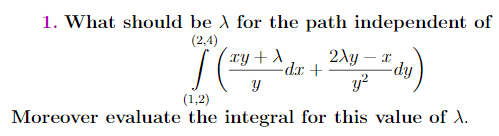I selected lambda as 4 (I actually don't know what it must be) and try to make clear to myself like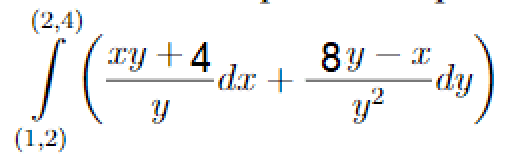these limits (1,2) and (2,4) is x and y locations I think :)
If I find an answer for part one of the integral following, I would apply this on another: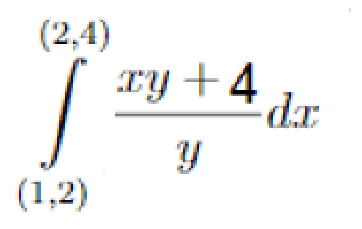My solution was :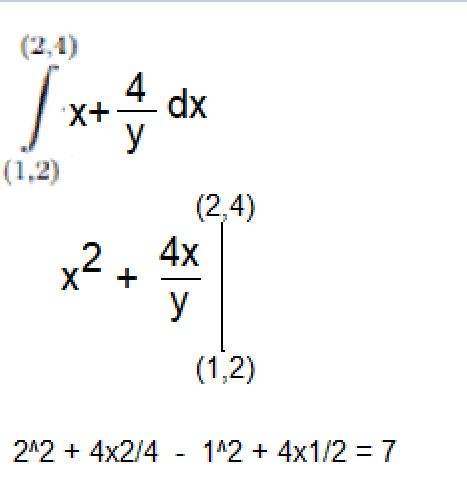I wonder whether it is correct. If it is not, how must I imagine?

#### Attachments

Homework Helper
Homework Statement:: Evaluate the integral below
Relevant Equations:: there is no equations(?)

I have a question like this;

View attachment 263435
I selected lambda as 4 (I actually don't know what it must be) and try to make clear to myself like
You must select lambda so as the integral is path-independent, that is the integrand is exact differential .
https://en.wikipedia.org/wiki/Exact_differential

requied
You must select lambda so as the integral is path-independent, that is the integrand is exact differential .
https://en.wikipedia.org/wiki/Exact_differential
I glanced the link and knew the drill. But I didn't find u -the scalar potential- of ## \ F = (\frac{xy+1}{y},\frac{2y-x}{y^2})##.(so λ =1) I'll really appreciate it if someone calculate the u. I think it is not such difficult to someone who knows the progress. The problem is I am learning these things recently, but it's just a homework we didn't learn the subject before 'corona' and the university teacher has just gave us. These subjects are for just this semester

Homework Helper
I glanced the link and knew the drill. But I didn't find u -the scalar potential- of ## \ F = (\frac{xy+1}{y},\frac{2y-x}{y^2})##.(so λ =1)
You know the partial derivatives of the desired potential function: ##\partial _x u = \frac{xy+1}{y}## and ##\partial _y u = \frac{2y-x}{y^2}##.
Integrate the partial derivatives; the first one with respect to x, the second one with respect to y. The integration "constant" is an y function in the first case, and an x function in the second case.
$$u=\int{\frac{xy+1}{y}dx} +f(y)$$
and
$$u=\int{\frac{2y-x}{y^2}dy} +g(x)$$
The two expressions for u must be identical: choose f(y) and g(x) accordingly.

requiedI chose second one and found the answer like this. Idk whether it is incorrect. So I'll try for the first one for the same logic, I'll see if it will get the same answer.

Edit: from first one, because of there is no natural logarithmic situation, we can't get ln2 or something

requied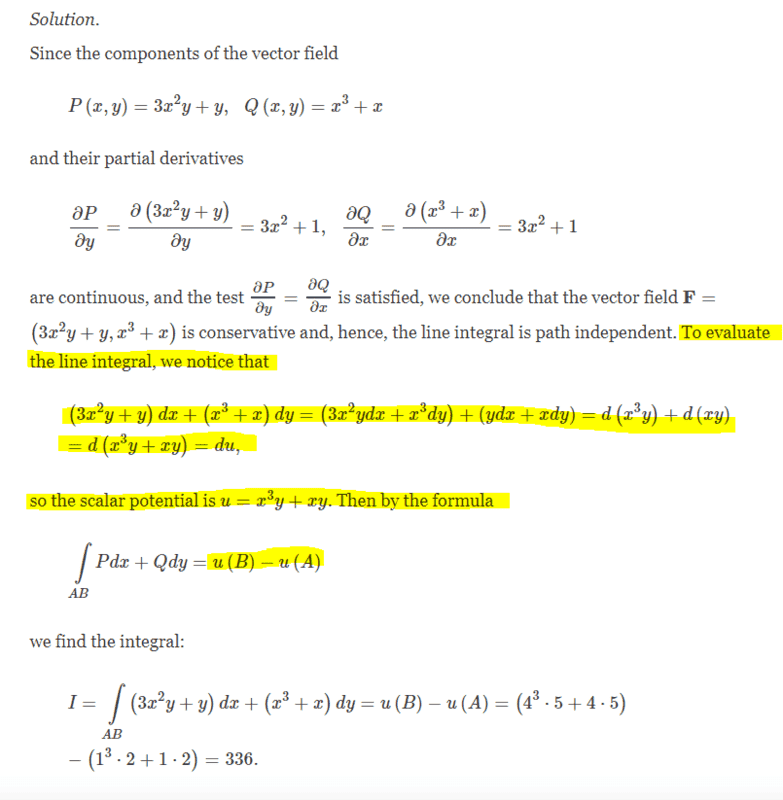Note it's not about my question, just an example.

Homework Helper
View attachment 264337
I chose second one and found the answer like this. Idk whether it is incorrect. So I'll try for the first one for the same logic, I'll see if it will get the same answer.

Edit: from first one, because of there is no natural logarithmic situation, we can't get ln2 or something
I do not understand what you did.You can get u(x,y) by integrating the second partial derivative with respect to y. Then determine the function g(x) by taking the partial derivative of u(x,y) with respect to x and make it identical to x+1/y.

requied
You can get u(x,y) by integrating the second partial derivative with respect to y. Then determine the function g(x) by taking the partial derivative of u(x,y) with respect to x and make it identical to x+1/y.
I did what you said at #9. Can you check out that for me?

Homework Helper
Gold Member
Your answer is not correct as you can verify that for your ##\phi##, ##\phi_x = P,~\phi_y = Q## fails. The problem is you have an extra ##\frac x y## in your answer. You really need to read the link I mentioned in post #7. In particular the last example.

Homework Helper
I found the potential function u like this:
View attachment 264368

I don't know why but it seems correct to me.
No, it is wrong. You just took the sum of the two integrals. Why?
When integrating, you have to include a constant. In this case, the integral with respect x has a "constant" that does depend on y. When integrating with respect y, the constant depends on x.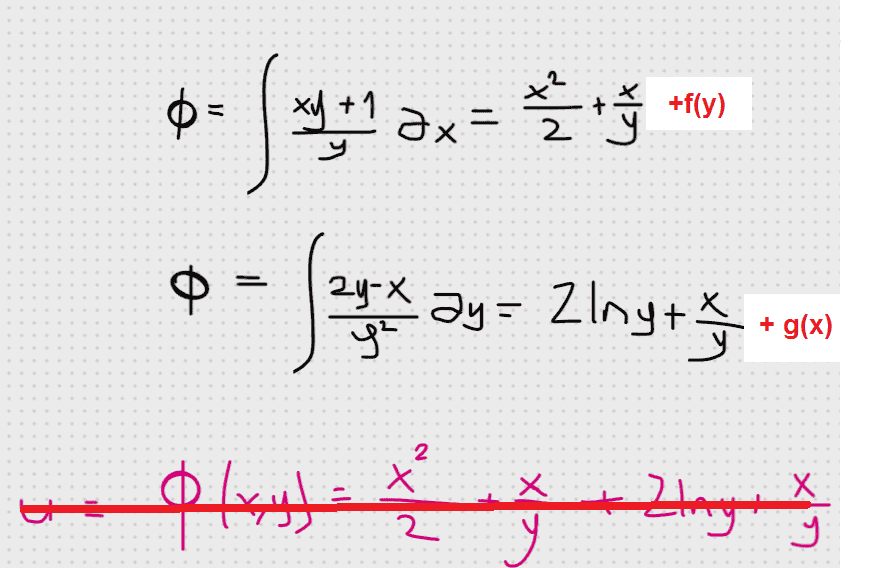The two ##\Phi(x,y) ## functions must be identical:
x^2/2+x/y+f(y)=2ln(y)+x/y+g(x)
What should be f(x) and g(y) so as the last equation is true?

•Delta2